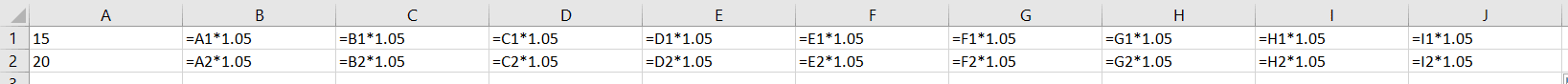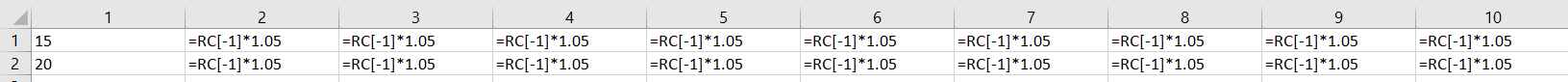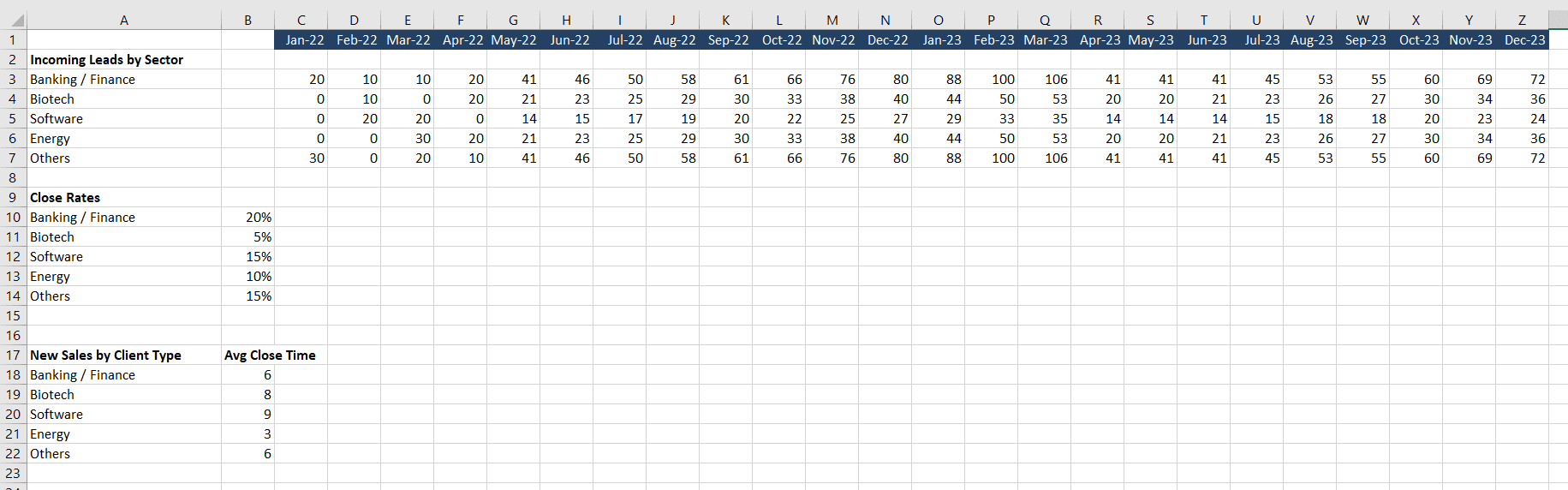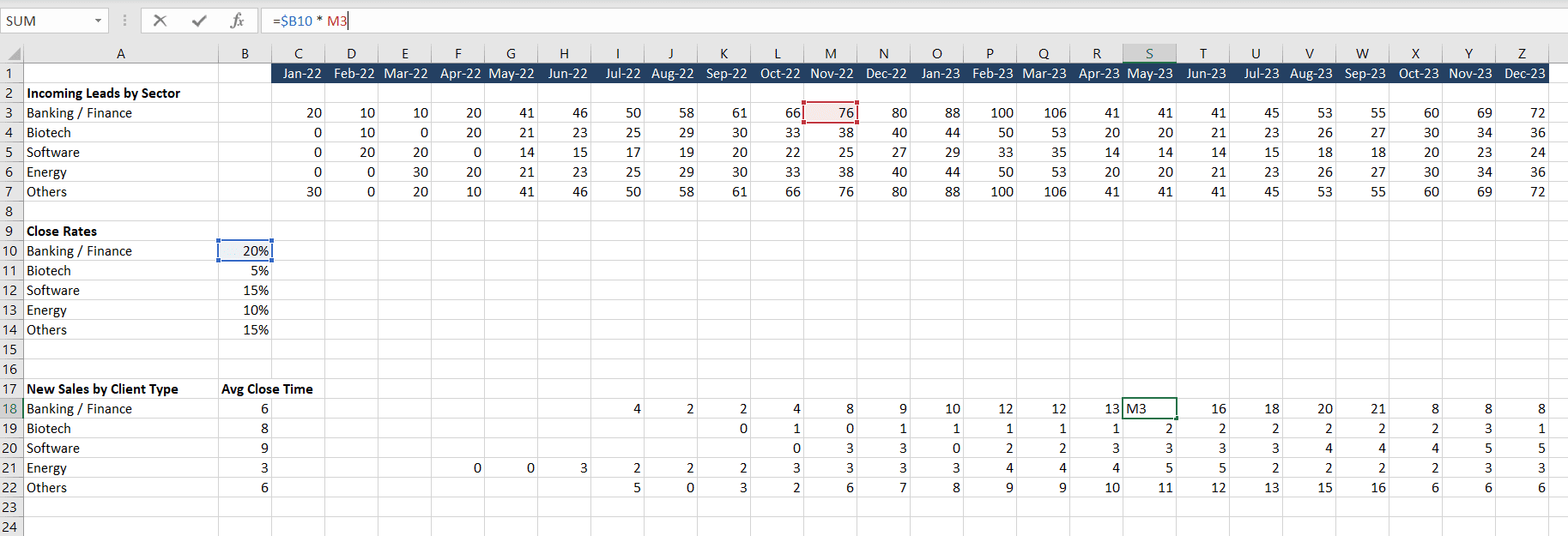A1-style notation for spreadsheets is intuitive and easy to work with. Columns are always identified by letters and rows always by numbers, so it’s hard to confuse which is which. The prevalence of using A1-notation is so thorough that sometimes it’s hard to imagine any other way to do it. But another way does exist.

In some circumstances, it makes more sense to use R1C1-style notation, which identifies the entire Excel grid by number. This becomes particularly useful when you need to perform some arithmetic on the column and row placement, such as moving down two rows and left one column.

## Connecting R1C1 to Past Experiences

R1C1 is just as intuitive as A1 notation once you’re accustomed to it. Many programmers already have plenty of experience with referencing ranges with R1C1 notation, because this is how the `.Cells` object works in Excel. It is very common to loop through a set of cells like this:

``````For i = 2 To 11
For j = 2 To 6
Cells(i, j).Value = Cells(i, j).Value * 1000
Next j
Next i``````

The code block above moves through a 10-by-5 grid and multiplies each number by 1000. References like this identify the row and the column by number, which is exactly what R1C1 does, albeit in a slightly different manner.

## The Power of R1C1

R1C1 notation take the same concept above and applies it to formulas. Instead of entering into cell B4 the formula `=B3*1.05` to increase B3 by 5%, we could write into B4 (R2C4) `=RC[-1]*1.05`, which references

1. the row offset by 0 (nothing)
2. the column offset by -1 (one to the left).

A consequence of this is that when dragging or autofilling, in A1 notation we’ll have formulas that look different in each cell but in R1C1 notation the formulas will all be exactly the same:Simple A1-Style Notation FormulasSimple R1C1-Style Notation Formulas

This visualization should be intuitive evidence of how powerful R1C1 notation can be: a single formula based on referential logic can generate complex tables. There is also a lot of flexibility in this referential style, which we’ll explore further.

## Types of R1C1 Reference

A1-style notation has a single type. You enter the column letter and the row number and that’s it. There are no other ways to make the reference. With R1C1, however, you can make absolute references, relative references, and mixed references.

### Absolute References

Absolute references in R1C1-style target a specific cell and they never change. For example, let’s add some absolute references to B2 and C2:

``````Range("B2").FormulaR1C1 = "=R2C1 * 1.05"      'set by A1 range notation
Cells(3, 2).FormulaR1C1 = "=R3C1 * 1.05"      'set by row/column cell notation``````

These two formulae reference R2C1 (cell A2) and R3C1 (cell A3) at all times, and they never change, even if you drag them across the sheet. It’s equivalent to typing `=\$A\$2 * 1.05` and `=\$A\$3 * 1.05` directly in the Excel cell.

### Relative References

Relative references are a little more interesting, because they let you leverage spatial logic. Simply use square brackets `[]` to make the R1C1 notation relative rather than absolute, just as we did earlier in the Power of R1C1 section.

• Positive numbers reference cells down ↓ or to the right →
• Negative numbers reference cells above ↑ or to the left ←
• No number (nothing ~=0) maintains the current row or column

The positive/negative difference is intuitive, at least for those of us who read top-to-bottom and left-to-right. A couple examples may help:

``````Range("B2").FormulaR1C1 = "=R[-1]C[-1]"         'refs A1, one row up (-1) and one column left (-1)
Range("B2").FormulaR1C1 = "=RC[-1]"          'refs A4, two rows down (+2) and one column left (-1)
Range("B2").FormulaR1C1 = "=RC"              'refs E2, zero row changes and three columns right (+3)
Range("B2").FormulaR1C1 = "=RC"           'refs E2, zero row changes and three columns right (+3)``````

Notice how similar this syntax is to the VBA Offset property. With these relative references, if you drag or autofill the formulas on the worksheet, you’ll get a the same spatial relations. The first formula above in B2 references A1, but if we put it into C3, it would reference B2. This contrasts with the absolute reference, which will always reference the same cell, no matter where it is on the sheet.

Make powerful macros with our free VBA Developer Kit

It’s easy to copy and paste a macro like this, but it’s harder make one on your own. To help you make macros like this, we built a free VBA Developer Kit and wrote the Big Book of Excel VBA Macros full of hundreds of pre-built macros to help you master file I/O, arrays, strings and more - grab your free copy below.

I'll take a free VBA Developer Kit

### Mixed References

These two styles can be combined, too. Simply add or exclude the square brackets to mix.

``````Range("B2").FormulaR1C1 = "=R1C[-1]"            'refs A1, but the row will always be 1
Range("B2").FormulaR1C1 = "=RC2"             'refs B4, but the column will never change (always B)``````

### Lock Rows and Lock Columns with R1C1

You may have noticed that for absolute references, the column or row never changes. This seems awfully similar to locking a row or column - and that is exactly what it does.

An absolute reference, such as `=R1C2 * 1.05` will always grow cell B1 by 5%, no matter where it is entered in the worksheet. Again, in the more familiar A1 notation, this formula would appear as `=\$B\$2 * 1.05`. These two formulas are identical, except one is in R1C1 notation and the other is in A1 notation. Let’s take a look at a couple more examples with locked rows and columns.

``````Range("B2").FormulaR1C1 = "=R1C[-1]*1.05"            'equivalent to A\$1 * 1.05, as the row is locked
Range("B2").FormulaR1C1 = "=RC2*1.05"             'equivalent to \$B4 * 1.05, as the column is locked``````

Note that when making absolute references, it doesn’t matter what your starting cell is. The absolute reference must be specified.

## Variable R1C1 References

Variables are a core component of programming. There isn’t much point to programming if some value isn’t varying.

The `Range.FormulaR1C1` inputs a formula into a cell, and the formula is written as a string in the VBA code. This means you can concatenate any numerical variable into the R1C1 formula using string concatenation.

This comes in handy when coupled with relative references, allowing you to target different cell distances based on variables.

``````numMonths = Range("A1")
Range("B2").FormulaR1C1 = "=R[-1]C[" & numMonths & "]*1.05"``````

Here, we place a formula in cell B2 that increases a value by 5%. The cell we’re referencing is in Row 1 (from `R[-1]`) and some column determined by the value numMonths in cell A1. If the number of months is 6, then the formula in B2 would be `=R[-1]C * 1.05` or, based on its initial cell B2, `=H1 * 1.05`, which is one row above B2 and 6 columns to the right.

## Modeling Variable Client Closing Times

I always like to give examples, and I recently had the opportunity to apply this type of variable R1C1 referencing at my full-time job (which is not actually programming).

I was building a financial model that included multiple client types and each type had a different length of time from lead-in to contract signing. The time to close greatly affects cash flows, because cash only flows in after the client signs. If a client drags out the process - quite common in my experience - cash could dry up, especially in a start-up environment. Averages and smoothing techniques do not apply because closing is a binary decision. Clients rarely pay before the contract is signed, and you can’t extrapolate future earnings to earlier dates.

I came up with three ways to build the model:

• use HLOOKUPs and a table
• use user-defined functions (UDFs)
• use R1C1 references

The first option used a complicated formula which would be difficult for investors and the financial team to parse. The second option required auditors, investors, and the financial team to be able to read VBA, which is, unfortunately, not a very common skill. The third option allowed me to make very clear references but still automate filling out the sheet.

To be transparent, we did end up using HLOOKUP, even though it was messy, because it allowed investors and auditors to easily perform what-if analyses with an .xlsx file instead of an .xlsm file, which are often blocked on internal bank computers. That said, for unsophisticated investors and financial teams, using R1C1 references populated by VBA is still an illustrative approach.

### The Setup

Our sheet was set up like in the image below (I’ve changed details for confidentiality). The top section is the number of expected leads, based on marketing, networking, etc. The next section is how many of the leads are expected to close. And the last section provides the expected close time (in months) and the actual number of clients.Client leads, close rates, and times

### The Code

Now for the code. The code essentially enters a formula in each cell in the range C18:Z22 that references the close rate and the client leads from a specific number of months ago.

``````Sub populateClientLeads()
Dim monthsToClose, currMonth, clientType As Integer

For clientType = 0 To 4  'iterates through all 5 client types

monthsToClose = Cells(18 + clientType, 2)   'grabs number of months to close for current client type

For currMonth = 0 To 23     'starts from 0, January 2022, but note the Cells reference must target the correct cell

If currMonth - monthsToClose < 0 Then       'move current month forward in time until close month makes sense (close must be greater than current month)
Do Until currMonth - monthsToClose = 0
currMonth = currMonth + 1
Loop
End If

Cells(18 + clientType, 3 + currMonth).FormulaR1C1 = "=R[-8]C2 * R[-15]C[-" & monthsToClose & "]"
'start in row 18 and move down for each successive client type
'start in column 3 and add the current month
'first R1C1 references the close rates, and notice how the column is locked
'second R1C1 looks 15 rows prior to get client leads for that client type then
'moves back a number of columns equal to the close time
Next currMonth
Next clientType

End Sub``````

This is a longer code block than we often post here, so here’s an example to help clarify.

Energy companies take 3 months to close, so to see how many energy companies actually sign contracts in June 2022, we should look at March 2022 leads, 3 months earlier than June.

Of course, we also need to incorporate the close rate (obviously not all leads close), and we must lock the close rate column, since this macro populates the entire range C18:Z22. The reason we reference the close rate with R1C1 style and explicitly add it to the formula is so that investors and auditors can inspect the formula themselves.

### The Result

The result is a fully-populated client sales section with a very simple-to-read formula. Investors and auditors can click on any cell in C18:Z22 and see exactly how it’s calculated. It is much more concise than trying to understand HLOOKUPs and VBA code (for the investors and auditors, at least).Populated sales with proper timing

In this screenshot, the cell S18 contains a formula that looks at the Banking / Finance close rate and checks the Banking / Finance leads 6 months prior to S18 (May 2023), which is November 2022. Manually adjusting each time the average close time changes, especially when doing what-if financial analyses, would be very cumbersome. With the macro, you change the average close time, click a macro button, and the formulas are all rewritten. Unfortunately for my application, that does require an .xlsm file, which couldn’t be run on the investors’ and auditors’ systems. I’m sure many of you can relate to that.

Now you can use R1C1-style notation and even lock columns and rows with it, use variable relative references, and apply cell-distance logic to formulas. R1C1 notation is easy to work with once you’re familiar with it and it makes any distance-based manipulations very easy in your VBA macros.

For more VBA tips and tricks like this, subscribe using the form below.